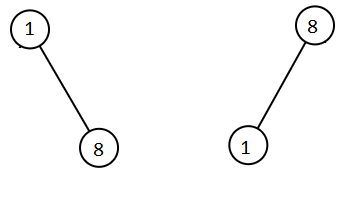Sep 05, 2020

# Day 5

A tree question that I knew.

## The Question

Given two binary search trees root1 and root2.

Return a list containing all the integers from both trees sorted in ascending order.

Example 1:Input: root1 = [2,1,4], root2 = [1,0,3]
Output: [0,1,1,2,3,4]


Example 2:

Input: root1 = [0,-10,10], root2 = [5,1,7,0,2]
Output: [-10,0,0,1,2,5,7,10]


Example 3:

Input: root1 = [], root2 = [5,1,7,0,2]
Output: [0,1,2,5,7]


Example 4:

Input: root1 = [0,-10,10], root2 = []
Output: [-10,0,10]


Example 5:Input: root1 = [1,null,8], root2 = [8,1]
Output: [1,1,8,8]


Constraints:

• Each tree has at most 5000 nodes.
• Each node's value is between [-10^5, 10^5].

## The Process

Note: My calculation of time complexity on this, is in no way, rigorous. A rigorous time complexity analysis is welcome, and so are more efficient solutions/improvements, although O(n1 + n2) lower-bounds all solutions’ runtime ( n1, n2 being the number of elements in in root1, root2), respectively. This is simply because you can’t do better, and at the very least, will have to visit each element.

Algorithm

• The ideas here are pretty simple. However, before reading on, I recommend that you look up inorder traversal and also solve the problem of merging two sorted lists. Read on only after you still have no idea on how to go about approaching this question.
• Note that you know the trees provided to us satisfy binary tree constraints, i.e. an inorder traversal/walk would yield elements in a sorted order. If you have a list in your hand, you can actually create a sorted list during your inorder walk/traversal.
• You also understand that eventually, you want a sorted list.
• Hence, would it be easy for us to first kind of flatten both the trees into sorted lists, and then merge these two sorted lists? Yes! * This is a rather straightforward question. Besides what the explanation covers, does anything else confuse you? Feel free to post that as a comment!

Time Complexity Time complexity here is very straightforward. You have two root nodes i.e. two trees. Call them T1 and T2. Let n1, n2 be the number of TreeNodes in T1 and T2, respectively. First, you do an inorder traversal of each of both T1 and T2, and get two sorted lists. This takes O(n1 + n2) since you visit each element in both trees exactly once. After this, you merge the two sorted lists i.e. another computation that costs O(n1 + n2) (since that’s the total number of elements in both the lists combined. Therefore, overall time complexity => O(n1 + n2).

## The Code

public List<Integer> getAllElements(TreeNode root1, TreeNode root2) {
List<Integer> list1 = inorder(root1, new ArrayList<>());
List<Integer> list2 = inorder(root2, new ArrayList<>());
return merge(list1, list2);
}
/**
* Provided a root node, this function returns a sorted list of the tree's elements using
* inorder traversal.
**/
public List<Integer> inorder(TreeNode root, List<Integer> list) {
if (root == null) {
return list;
}

if (root.left == null && root.right == null) {
return list;
}

inorder(root.left, list);
inorder(root.right, list);

return list;
}

/**
* Given two sorted lists, list1 and list2, we create a sorted output list that is a union
* of all the elements in list1 and list2.
**/
public List<Integer> merge(List<Integer> list1, List<Integer> list2) {
List<Integer> res = new ArrayList<Integer>(list1.size() + list2.size());

int i = 0;
int j = 0;

while (i < list1.size() && j < list2.size()) {
if (list1.get(i) <= list2.get(j)) {
i++;
continue;
}

if (list1.get(i) > list2.get(j)) {
j++;
}
}

if (i < list1.size()) {
for (int remaining = i; remaining < list1.size(); remaining++) {
}